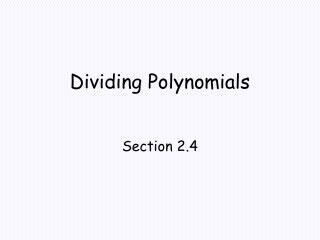DownloadDownload PresentationDividing Polynomials

# Dividing Polynomials

Télécharger la présentation## Dividing Polynomials

- - - - - - - - - - - - - - - - - - - - - - - - - - - E N D - - - - - - - - - - - - - - - - - - - - - - - - - - -
##### Presentation Transcript

1. Dividing Polynomials Section 2.4

2. Objectives • Divide two polynomials using either long division or synthetic division. • Use the Factor Theorem to show that x-c is a factor of a polynomial. • Use the Remainder Theorem to evaluate a polynomial at a given value.

3. Vocabulary • quotient • remainder • dividend • divisor

4. Division Algorithm If f (x) and d (x) are polynomials, with d(x) ≠ 0, and the degree of d(x) is less than or equal to the degree of f (x), then there exists unique polynomials q(x) and r(x) such that f(x) = d(x) · q(x) + r(x) The remainder, r(x), equals 0 or it is of degree less than the degree of d(x). If r(x) = 0, we say that d(x) divides evenly into f(x) and that d(x) and q(x) are factors of f(x).

5. Find the quotient and remainder using long division.

6. Find the quotient and remainder using long division.

7. Find the quotient and remainder using synthetic division.

8. Remainder Theorem If the polynomial f (x) is divided by x – c, then the remainder f (c).

9. Use synthetic division to show that x = 6 is a root of

10. Factor Theorem • Let f (x) be a polynomial • If f(c) = 0, then x – c is a factor of f (x). • If x – c,is a factor of f(x), then f(c) = 0.